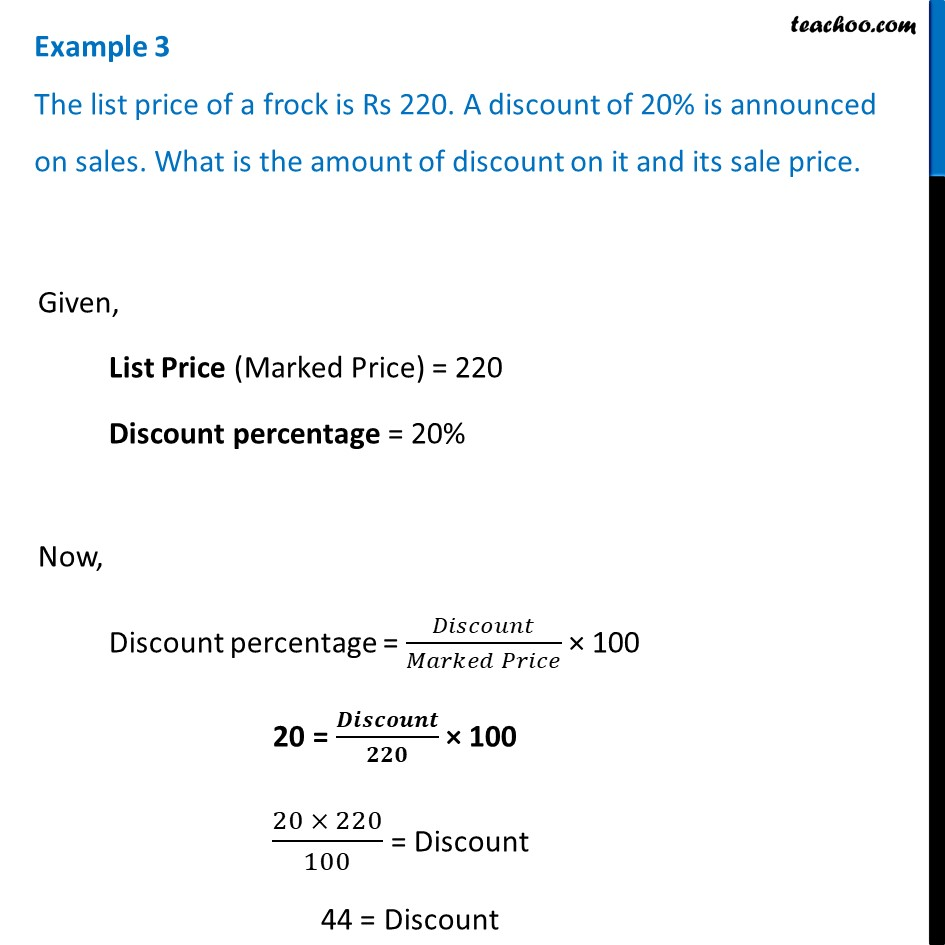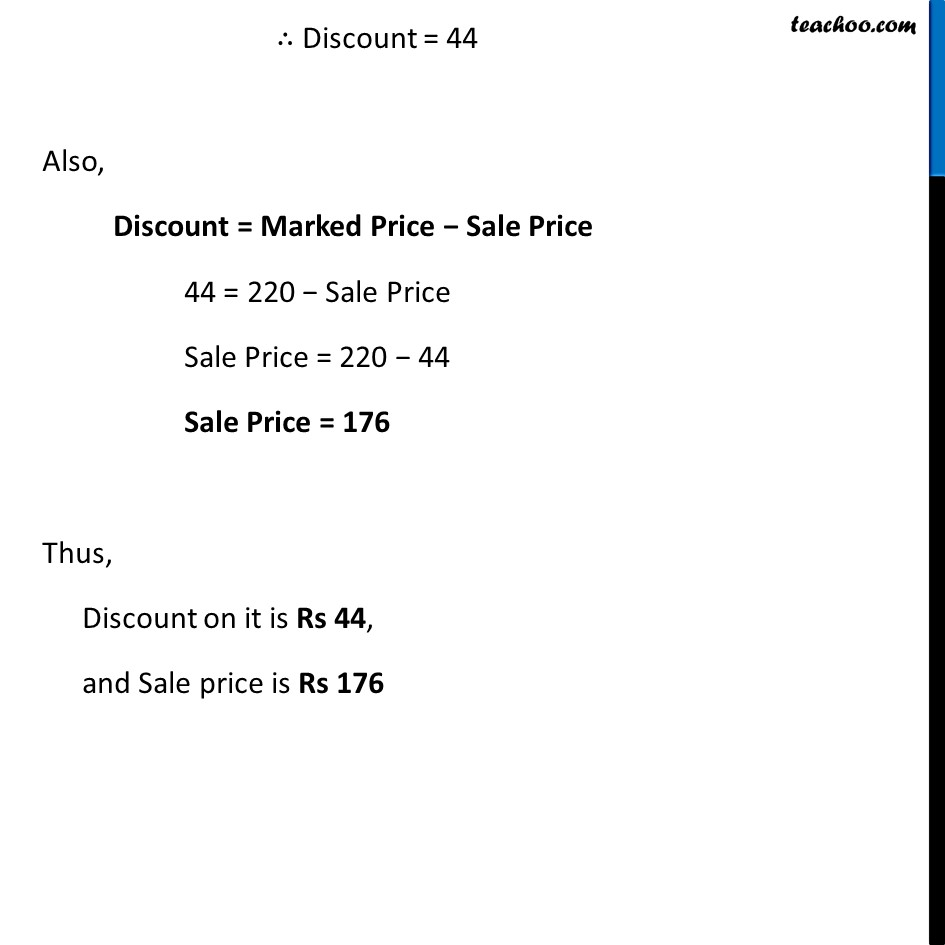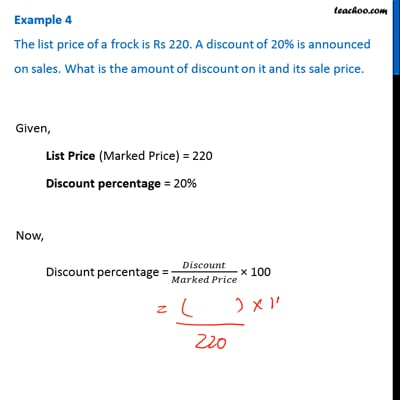Examples

Chapter 7 Class 8 Comparing Quantities
Serial order wiseThis video is only available for Teachoo black users

Learn in your speed, with individual attention - Teachoo Maths 1-on-1 Class

### Transcript

Example 3 The list price of a frock is Rs 220. A discount of 20% is announced on sales. What is the amount of discount on it and its sale price.Given, List Price (Marked Price) = 220 Discount percentage = 20% Now, Discount percentage = 𝐷𝑖𝑠𝑐𝑜𝑢𝑛𝑡/(𝑀𝑎𝑟𝑘𝑒𝑑 𝑃𝑟𝑖𝑐𝑒) × 100 20 = 𝑫𝒊𝒔𝒄𝒐𝒖𝒏𝒕/𝟐𝟐𝟎 × 100 (20 × 220)/100 = Discount 44 = Discount ∴ Discount = 44 Also, Discount = Marked Price − Sale Price 44 = 220 − Sale Price Sale Price = 220 − 44 Sale Price = 176 Thus, Discount on it is Rs 44, and Sale price is Rs 176